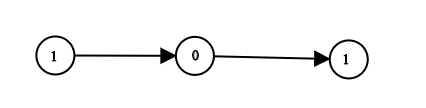##### Welcome to Subscribe On Youtube

Formatted question description: https://leetcode.ca/all/1290.html

# 1290. Convert Binary Number in a Linked List to Integer (Easy)

Given head which is a reference node to a singly-linked list. The value of each node in the linked list is either 0 or 1. The linked list holds the binary representation of a number.

Return the decimal value of the number in the linked list.

Example 1:Input: head = [1,0,1]
Output: 5
Explanation: (101) in base 2 = (5) in base 10


Example 2:

Input: head = 
Output: 0


Example 3:

Input: head = 
Output: 1


Example 4:

Input: head = [1,0,0,1,0,0,1,1,1,0,0,0,0,0,0]
Output: 18880


Example 5:

Input: head = [0,0]
Output: 0


Constraints:

• The Linked List is not empty.
• Number of nodes will not exceed 30.
• Each node's value is either 0 or 1.

Related Topics:

## Solution 1.

// OJ: https://leetcode.com/problems/convert-binary-number-in-a-linked-list-to-integer/
// Time: O(N)
// Space: O(1)
class Solution {
public:
int ans = 0;
ans = (ans << 1) + head->val;
}
return ans;
}
};


Java

• /**
* public class ListNode {
*     int val;
*     ListNode next;
*     ListNode(int x) { val = x; }
* }
*/
class Solution {
int decimalValue = 0;
while (temp != null) {
decimalValue <<= 1;
decimalValue += temp.val;
temp = temp.next;
}
return decimalValue;
}
}

• // OJ: https://leetcode.com/problems/convert-binary-number-in-a-linked-list-to-integer/
// Time: O(N)
// Space: O(1)
class Solution {
public:
int ans = 0;
return ans;
}
};

• # Definition for singly-linked list.
# class ListNode:
#     def __init__(self, val=0, next=None):
#         self.val = val
#         self.next = next
class Solution:
def getDecimalValue(self, head: ListNode) -> int:
ans = 0